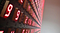# What is an Integer

## A basic data type, but what is it?

A simple question. Some might say “whole number”, but that only tells half the story. Read on for more information.Photo by Franck V. on Unsplash

Difficulty: Beginner | Easy | Normal | Challenging

# Prerequisites:

• None, although some knowledge of Binary would be useful (Guide: HERE)

# Terminology

Data types: A representation of the type of data that can be processed, for example Integer or String

Integer: A number that has no fractional part, that is no digits after the decimal point

Two’s Complement: The complement of a binary number with respect to 2^n

# Integers:

## A simple definition:

An `Integer` is a whole number. This means that 0, 3, 10, 12932 and -12 are all `Integers`, meaning that Integers can be both positive and negative numbers.

However, since `Integers` are whole numbers this means that the lack decimal places.

`Integers` are commonly used in programming languages, and we will see some ways that they are used below.

## Integer Arithmetic

Integers can be used with multiplication, division addition and subtraction. These actually work as you would expect:

1+3 = 4

2 * 5= 10

19–3 = 16

However, Integer division is a little different. Since `Integers` don’t have decimal places usually the result is truncated to produce an `Integer`.

That is,

25 / 2 = 12

# Uses in programming languages

Within programming languages `Integers` are extremely common `data types` and here are some common uses:

## Incrementing Integers in a for loop

A common use for an `Integers` is for an index in a for loop

Java: For loop

`for (int i = 1; i < 5; i++) {    System.out.println(i);}`

JavaScript: For loop

`var i;for (i = 0; i < 5; i++) {    console.log(i)}`

Python: For loop

`for i in range(0, 3):    print(i)`

Swift: For loop

`for i in 1...5 {    print(i)}`

# Memory usage of Integers

`Integers` are typically 4-bytes and can store both positive and negative numbers, although this varies in particular programming languages.

Since 4 bytes is equivalent to 32-bit numbers from 0 to 4294967295

The `Integers` 1 to 4 could be stored in the following binary pattern

Now it is possible that the order is reversed:

Negative values

In practice it is possible to store negative numbers by using the first bit as a sign bit.

The first bit can be 0 for positive numbers and 1 for negative numbers,

This action limits the numbers that can be stored to between -2147483648 and 2147483647.

Larger Integer values

On 64-bit machines `Integers` may be stored in 8-bytes of memory.

# Conclusion:

`Integers` are particularly important in programming, as they are the usual way to store whole numbers, and that is something that invariably you will want to do.

If you want to store larger `Integers` you might think about using larger memory (for example use double the memory), storing `Integers` as floating numbers (possibly introducing precision inaccuracies) or even using arbitrary precision arithmetic which uses a flexible amount of memory per `Integer` but takes longer to produce the answer to calculations.

# Extend your knowledge

• Integers on wikipedia (Link)
• To simplify arithmetic Two’s Complement is often used to store numbers (Link)

# The Twitter contact:

Any questions? You can get in touch with me here

stevecurtis.me